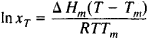# Shreder Equation

The following article is from The Great Soviet Encyclopedia (1979). It might be outdated or ideologically biased.

## Shreder Equation

a mathematical equation relating the solubility of a crystalline body xT at a temperature T (in °K) to its heat of melting ΔHm (in cal/mole) and melting point Tm:where R is the gas constant; ΔHm is taken as constant in the interval Tm–T. The rigorous application of the Shreder equation is limited to ideal solutions. By constructing curves for the temperature dependence of the solubility of a solid phase of each of the components of a binary system using the Shreder equation, it is possible to find the eutectic point and to obtain the solubility diagram.

The Shreder equation was derived in 1890 by I. F. Shreder (Schröder). It is also known as the Shreder logarithm and the Shreder-Le Chätelier equation. (H. Le Chätelier previously had obtained an equation for solubility as a function of temperature in differential form and, using this expression, derived an equation similar to the Shreder equation in 1894.)

### REFERENCE

Kipnis, A. Ia. Razvitie khimicheskoi termodinamiki v Rossii. Moscow-Leningrad, 1964.

M. KH. KARAPET’IANTS

The Great Soviet Encyclopedia, 3rd Edition (1970-1979). © 2010 The Gale Group, Inc. All rights reserved.
Site: Follow: Share:
Open / Close